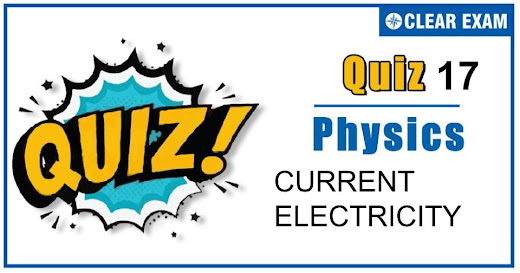## [LATEST]\$type=sticky\$show=home\$rm=0\$va=0\$count=4\$va=0

As per analysis for previous years, it has been observed that students preparing for NEET find Physics out of all the sections to be complex to handle and the majority of them are not able to comprehend the reason behind it. This problem arises especially because these aspirants appearing for the examination are more inclined to have a keen interest in Biology due to their medical background. Furthermore, sections such as Physics are dominantly based on theories, laws, numerical in comparison to a section of Biology which is more of fact-based, life sciences, and includes substantial explanations. By using the table given below, you easily and directly access to the topics and respective links of MCQs. Moreover, to make learning smooth and efficient, all the questions come with their supportive solutions to make utilization of time even more productive. Students will be covered for all their studies as the topics are available from basics to even the most advanced. .

Q1. The equivalent resistance between points A and B of an infinite network of resistances each of 1Ω connected as shown in figure, is?
•  Infinite
•  Zero
•
•  (1+√5)/2Ω
Solution
The x be the total resistance of infinite network of resistance connected to points A and B. Therefore the addition of one step of resistances in the infinite network of resistances will not change the total resistance x of the network. Therefore equivalent circuit will be as shown in figure. Then total resistances between A’ and B’ is x given by x=1+(1+x)/(1+x)=(1+2x)/(1+x) or x+x2=1+2x
or x2-x-1=0 or x=(1±√5)/2=(1+√5)/2 Since negative value of R is not possible

Q2. By mistake a voltmeter is connected in series and an ammeter is connected in parallel with a resistance in an electrical circuit. What will happen to the instrument?
•  Voltmeter is damaged
•  Ammeter is damaged
•  Both are Damaged
•  None is damaged
Solution
Due to high resistance of voltmeter, connected in series, the effective resistance of circuit will increase and hence the current in circuit will decrease. Due to which the ammeter and voltmeter will not be damaged..

•  8.31 A
•  6.82 A
•  4.92 A
•  2 A
Solution
6Ω and 6Ω are in series, so effective resistance is 12Ω which is in parallel with 3Ω, so 1/R=1/3+1/12=15/36 ⇒R=36/15 ∴I=V/R=(4.8×15)/36=2A

Q4. Specific resistance of copper, constantan and silver are 1.78×10-8,39.1×10-8 and 10-8 Ω-m respectively. Which of these is the best conductor of heat and electricity?
•  Copper
•  Constantan
•  Silver
•  All of them
Solution
The best conductor of electricity is one whose resistance is least. AsR=ρl/A.Therefore for the given value of land A,R∝ρ. Hence, silver is the best conductor of heat and electricity

Q5. Two bulbs X and Y having same voltage rating and of power 40 W and 60 W respectively are connected in series across a potential difference of 300 V, then?
•  X will glow brighter
•  Resistance of Y will be greater than X
•  Heat produced in Y will be greater than X
•  Voltage drop in X will be greater than Y
Solution
Resistance of bulb R=V2/P R∝1/P Here PX=40 W,and PY=60 W ∴ RX>RY So, potential drop across bulb X ie, of 40 W bulb will be greater and it will glow brighter.

Q6. A current passing through a copper voltmeter deposits 0.002 kg of copper on cathode plate in 100 min. If there are 1025 copper atoms in one kg of copper, the electric charge delivered to cathode by Cu++ ions per second will be?
•  0.53 C
•  0.71 C
•  1.06 C
•  10.06 C
Solution
Charge delivered to cathode per second =(0.002×1025×2×1.6×10-19)/(100×60) =1.06 C

Q7. The resistance of an ideal ammeter is?
•  Infinite
•  Very high
•  Small
•  Zero
Solution
Zero

Q8. A potential difference of V is applied at the ends of a copper wire of length l and diameter d. On doubling only d, the drift velocity?
•  Becomes two times
•  Becomes half
•  Does not change
•  Becomes one-fourth
Solution
vd=I/nAl=1/nAe×V/R =1/nAe×V/((ρl/A))=V/neρl As vd is independent of area of cross-section hence drift velocity will not change, when diameter is doubled

Q9. Consider a cylindrical element as shown in the figure. Current flowing through element is I and resistivity of material of the cylinder is ρ. Choose the correct option out the following?
•  Power loss is second half is four times the power loss in first half
•  Voltage drop in first is twice of voltage drop in second half
•  Current density in both halves are equal
•  Electric field in both halves is equal
Solution

VAB=I.RAB=(I.ρ.LAB)/A1 =(I.ρ(L/2))/(π(2r)2 )=(I ρ.(L/2))/(π4r2 ) VAB=(I ρ.L)/(8πr2 ) VBC=I.RBC=(I.ρ.L)/A2 =(I.ρ.L/2)/(π(r2))=(I.ρ.L)/(2πr2 )⇒VAB/VBC =((I.ρ.L)/(8πr2 ))/((I.ρ.L)/(2πr2 ))=2/8=1/4 VAB=VBC/4 Now for power loss PAB=V(A.B).I PBC=VBC.I PAB/PBC =VAB/VBC =1/4⇒VAB=PBC/4

Q10. A current of 3 amp. flows through the 2Ω resistor shown in the circuit. The power dissipated in the 5Ω resistor is?

•  1 watt
•  5 watt
•  4 watt
•  2 watt
Solution
Current flowing through 2Ω resistance is 3A, so P.D. across it is 3×2=6V Current through the bottom line =6/(1+5)=1A ∴ Power dissipated in 5Ω resistance is P=i2 R=12×5=5W## Want to know more

Please fill in the details below:

## Latest NEET Articles\$type=three\$c=3\$author=hide\$comment=hide\$rm=hide\$date=hide\$snippet=hide

Name

ltr
item
BEST NEET COACHING CENTER | BEST IIT JEE COACHING INSTITUTE | BEST NEET & IIT JEE COACHING: Current Electricity Quiz 17
Current Electricity Quiz 17